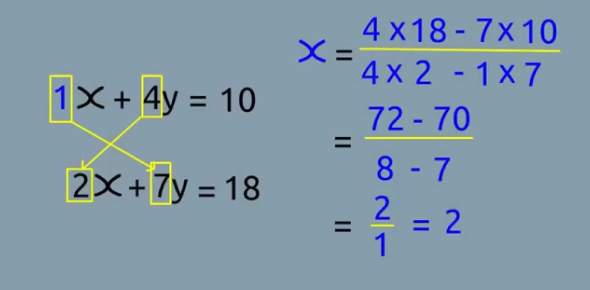# Simultaneous Equations Trivia Quiz!

61 Questions | Attempts: 905
ShareSettingsWhat are simultaneous equations? How easily can you solve simultaneous equations? We have this trivia quiz based on that to check if you know about simultaneous equations and coordinate forms. In mathematics, a simultaneous equation is an algebraic equation that involves two or more quantities (x and y) related to each other in two or more equations. You need to solve equations for the variables x and y. Do you think you can do so? Play the quiz and check now.Back to top